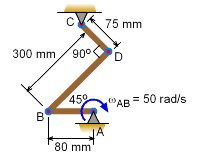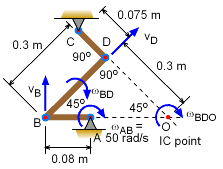Ch 5. Rigid Body General Motion Multimedia Engineering Dynamics Fixed Axis Rotation Plane Motion Velocities Zero Velocity Point Plane Motion Accelerations Multiple Gears Rot. Coord. Velocities Rot. Coord. Acceleration
 Chapter - Particle - 1. General Motion 2. Force & Accel. 3. Energy 4. Momentum - Rigid Body - 5. General Motion 6. Force & Accel. 7. Energy 8. Momentum 9. 3-D Motion 10. Vibrations Appendix Basic Math Units Basic Equations Sections Search eBooks Dynamics Fluids Math Mechanics Statics Thermodynamics Author(s): Kurt Gramoll ©Kurt GramollDYNAMICS - EXAMPLEThree Link Mechanism Example The rod AB rotates at ωAB= 50 rad/s about the fixed point A. The rod, CD is pinned at point C. Determine the angular velocity of the rod CD at the instant shown. SolutionPoint of Zero-Velocity Method This could be solved using relative motion equations (vectors) as explained previous section, General Plane Motion: Velocities. However, for many velocity plane motion problems, using the instantaneous center of zero velocity (IC) can simplify the problem. The IC point will be at the intersection of the two lines perpendicular to the velocities of point B and D. For this instant, the triangle OBD is rotating about point O. The distances DO and BO are,      rOD = rBD tan45 = 0.3 (1) = 0.3 m      rOB = rBD / cos45 = 0.3 / 0.7071 = 0.4243 m The velocity of point B is,       vB = ωAB rAB = 50 (0.08) = 4 m/s Thus, the angular velocity of BD is,      ωBD = ωBDO = vB/rBO = 4 / 0.4243 = 9.428 rad/s The velocity of point D can now be determined,      vB = ωBD rOD = 9.428 (0.3) = 2.828 mr/s Finally, the angular velocity of CD is      ωCD = vB / rCD = 2.828 / 0.075 = 37.71 ωCD = 37.71 rad/s

Practice Homework and Test problems now available in the 'Eng Dynamics' mobile app
Includes over 400 problems with complete detailed solutions.
Available now at the Google Play Store and Apple App Store.